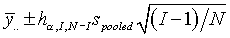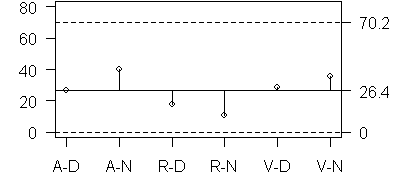# Analysis of Means calculations

## 2007-03-02

Analysis of Means (ANOM) are available for a wide range of data sets, and it is impossible to summarize all the applications of ANOM here. The goal on this web page is to illustrate a few of the calculations.

The simplest application of ANOM is a balanced single factor study. A single factor represents measurements done across individuals or sometimes machines, or other conditions). Let I represent the number of individuals being studied and let N represent the total number of observations. If there are m observations per individual, then N=mI.

For each individual, calculate a mean and a variance. The formula for upper and lower decision limits in this setting iswhereAs a classroom exercise, a group of three volunteers (labeled A, R, and V to protect their anonymity) were asked to take turns hitting a target with a hand launched foam rocket.

### Shooting at the target### Measuring the resultSince the group was small, I asked each team member to shoot twice with their dominant hand and twice with their non-dominant hand. For each shot, the distance from the target was measured in centimeters. Here is the data (D=dominant hand, N=non-dominant hand).

A-D 14 39
A-N 60 20
R-D 26  9
R-N  9 12
V-D 36 21
V-N 53 18


The means, variances, and standard deviations are:

    Mean Var   Stdev
A-D 26.5 312.5 17.68
A-N 40.0 800.0 28.28
R-D 17.5 144.5 12.02
R-N 10.5   4.5  2.12
V-D 28.5 112.5 10.61
V-N 35.5 612.5 24.75


The overall mean is 26.42 and the pooled standard deviation is 18.20. The ANOM limits are

26.4 - 3.724 * 18.2 * sqrt(5/12) = -17.3 (round this to zero)
26.4 + 3.724 * 18.2 * sqrt(5/12) =  70.1


Here is a graph of the results:You can find an earlier version of this page on my old website.